##### Actions

of a Lie group

The linear action on the Lie algebraof the Lie group, denoted by, that is defined as follows: Each elementofinduces an inner automorphism of the Lie groupby the formula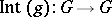,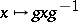. Its differential,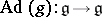, gives an automorphism of the Lie algebra. The resulting linear representationis called the adjoint representation of(cf. also Adjoint representation of a Lie group).

The kernel of the adjoint representation,, contains the centreof(cf. also Centre of a group), and coincides withifis connected. The imageis called the adjoint group; it is a Lie subgroup of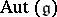, the group of all automorphisms of the Lie algebra.

The differential of the adjoint representationgives rise to a linear representation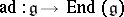of the Lie algebra(cf. also Representation of a Lie algebra). It is given by the formula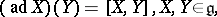and is called the adjoint representation of(cf. also Adjoint representation of a Lie group). The kernel,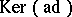, coincides with the centre of the Lie algebra. The image,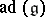, forms a subalgebra of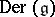, the Lie algebra of all derivations of(cf. Derivation in a ring). Ifis a semi-simple Lie algebra (see Lie algebra, semi-simple), thenand. An opposite extremal case is whenis Abelian; in this case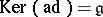and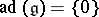.

If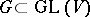is a linear group acting on a vector space, then one can regard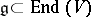and the adjoint representation can be written in terms of matrix computation: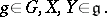The adjoint orbit through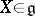(see Orbit) is defined to be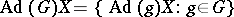; it is a submanifold of.

Adjoint orbits for reductive Lie groupshave been particularly studied. The adjoint orbit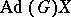is called a semi-simple orbit (respectively, a nilpotent orbit) ifis a semi-simple (respectively, nilpotent) endomorphism of. The setis an algebraic variety in, called the nilpotent variety. It is the union of a finite number of nilpotent orbits. On the other hand,is a closed set inif and only if it is a semi-simple orbit. A semi-simple orbitis called an elliptic orbit (respectively, a hyperbolic orbit) if all eigenvalues of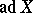are purely imaginary (respectively, real). Any elliptic orbit carries a-invariant complex structure, while any hyperbolic obit carries a-invariant paracomplex structure.

Ifis compact, then all adjoint orbits are elliptic. For example, if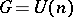(a unitary group), then each adjoint orbit is biholomorphic to a generalized flag variety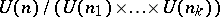, for some partition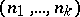of, and vice versa (cf. also Flag space; Algebraic variety).

One writes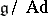for the set of all adjoint orbits. The classical theory of the Jordan normal form of matrices (as well as the theory of other normal forms of matrices; cf. also Normal form) can be interpreted as the classification of, for. Ifis a connected compact Lie group, thenis bijective to, the set of all orbits in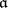of the Weyl group, whereis a maximal Abelian subspace of. This reduction is important in the Cartan–Weyl theory of the classification of irreducible representations, as well as their characters, for a compact Lie group (cf. also Lie group, compact; Irreducible representation).

Bi-invariant tensors on a Lie groupcan be described in terms of invariants under the adjoint action. For example, the left-invariant measure on a connected Lie groupis also right invariant if and only if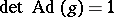for any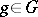. Such a Lie group is called unimodular (cf. Haar measure). This is the case ifis nilpotent or reductive.

Let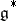be the dual vector space of. The contragredient representation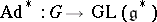of the adjoint representation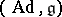is called the co-adjoint representation. There is a close connection between irreducible unitary representations ofand co-adjoint orbits (see Orbit method).

How to Cite This Entry: